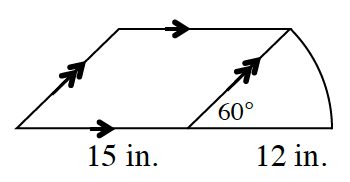### Home > INT2 > Chapter 9 > Lesson 9.2.2 > Problem9-71

9-71.A sector is attached to the side of a parallelogram, as shown in the diagram at right. Calculate the area and perimeter of the figure.

Remember that the straight sides of a sector are equal to each other and also the radius of the circle.

You can find the height of parallelogram by using $30^\circ-60^\circ-90^\circ$ triangle side identities.

$\text{Perimeter}≈ 66.6 \text{ in.}$